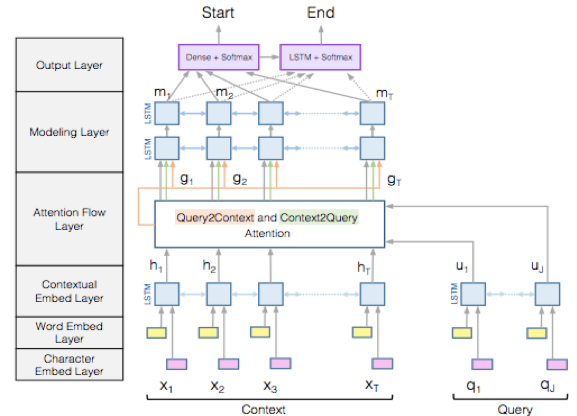# 机器阅读理解（machine comprehension）

1 输入编码模块。
2 context内容和query内容交互模块
3 输出预测模块1 输入模块：

2 交互模块：

context-to-query Attention：

query-to-context Attention：

3 输出模块

pstart=softmax(WTpstart[G;M])$p^{start}=softmax(W^T_{p_{start}}[G;M])$，其中WTpstart$W^T_{p_{start}}$是一个10d$10d$的向量，随着模型一起训练。

pend=softmax(WTpend[G;M2])$p^{end}=softmax(W^T_{p_{end}}[G;M^2])$，同样的WTpend$W^T_{p_{end}}$是一个10d$10d$的向量，随着模型一起训练。

L(θ)=1NNilog(pstartxi)+log(pendxi)$L(\theta)=-\frac{1}{N}\sum_{i}^N log(p_{x_i}^{start})+log(p_{x_i}^{end})$